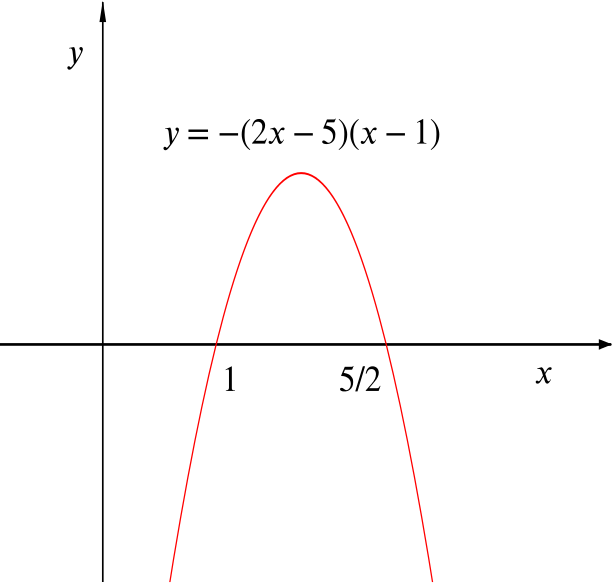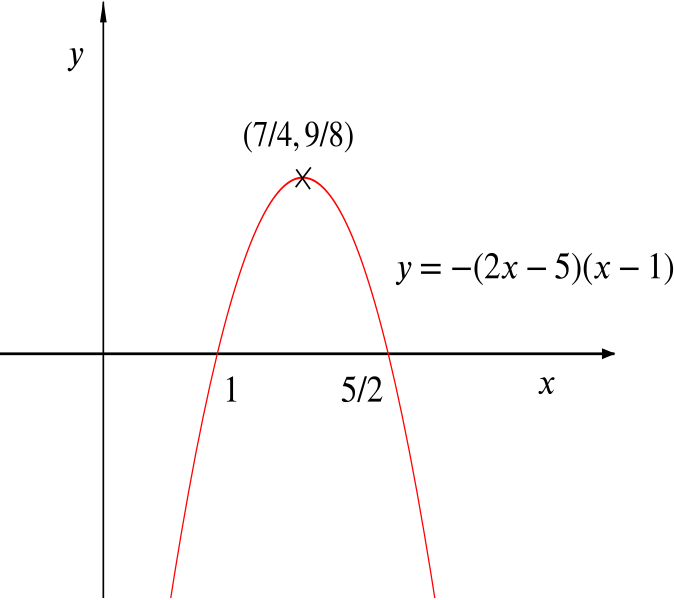Review question

# When is one of these functions greater than the other? Add to your resource collection Remove from your resource collection Add notes to this resource View your notes for this resource

Ref: R6008

## Solution

Functions $f$ and $g$ are defined by \begin{align*} f&:x\mapsto x(x-1), \\ g&:x\mapsto (x-1)(3x-5), \end{align*}

where $x\in \mathbb{R}$ in each case.

1. Find the solution set, $S$, of the inequality $f(x)\ge g(x).$
We want to find $x$ so that \begin{align*} & x(x-1)\ge (x-1)(3x-5) \\ \iff & x^2-x \ge 3x^2-8x+5 \\ \iff & 2x^2-7x+5\le 0 \\ \iff & (2x-5)(x-1)\le 0. \end{align*}

By considering the graph of $y=(2x-5)(x-1)$, we see that the solution is $1\le x\le \tfrac{5}{2}.$

1. Sketch the graph of $y=f(x)-g(x)$ for $x\in S$, and state the greatest and least values of $f(x)-g(x)$ for $x\in S$.

Using our working from above, $f(x)-g(x)=-(2x-5)(x-1)$, so the graph of $y = f(x) - g(x)$ looks like this:The graph of $y=f(x)-g(x)$

Since $S$ is the set of $x$ such that $f(x)-g(x)\ge 0$, the minimum value of $f(x)-g(x)$ on $S$ must be zero, when $x = 1$ or $\dfrac{5}{2}$.

To find the maximum, we can complete the square: \begin {align*} -2x^2+7x-5 &= -2\left(x-\frac{7}{4}\right)^2+\frac{49}{8}-5\\ &=-2\left(x-\frac{7}{4}\right)^2+\frac{9}{8}. \end {align*}

Since $2\left(x-\frac{7}{4}\right)^2 \ge 0$, we have $-2x^2+7x-5\le \tfrac{9}{8}$, so the maximum value of $f(x)-g(x)$ on $S$ is $\tfrac{9}{8}$, and this is attained at $x=\frac{7}{4}$. So we can sketch $f(x)-g(x)$ on $S$.

Alternatively we can say that $y = f(x) - g(x)$ has its maximum when $x = \dfrac{1 + \dfrac{5}{2}}{2}$, or $\dfrac{7}{4}$.

Now $y = \dfrac{9}{8}$ when $x=\dfrac{7}{4}.$The graph of $y=f(x)-g(x)$ on $S$